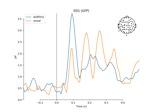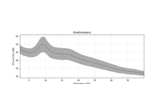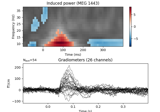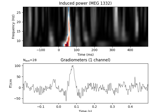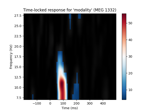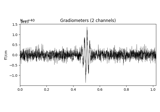# mne.time_frequency.tfr_morlet¶

mne.time_frequency.tfr_morlet(inst, freqs, n_cycles, use_fft=False, return_itc=True, decim=1, n_jobs=1, picks=None, zero_mean=True, average=True, output='power', verbose=None)[source]

Compute Time-Frequency Representation (TFR) using Morlet wavelets.

Same computation as `tfr_array_morlet`, but operates on `Epochs` objects instead of `NumPy arrays`.

Parameters
inst

The epochs or evoked object.

freqs`ndarray`, shape (n_freqs,)

The frequencies in Hz.

n_cycles`float` | `ndarray`, shape (n_freqs,)

The number of cycles globally or for each frequency.

use_fftbool, default `False`

The fft based convolution or not.

return_itcbool, default `True`

Return inter-trial coherence (ITC) as well as averaged power. Must be `False` for evoked data.

decim`int` | `slice`, default 1

To reduce memory usage, decimation factor after time-frequency decomposition. If `int`, returns tfr[…, ::decim]. If `slice`, returns tfr[…, decim].

Note

Decimation may create aliasing artifacts.

n_jobs`int`

The number of jobs to run in parallel (default 1). Requires the joblib package.

picks

The indices of the channels to decompose. If None, all available good data channels are decomposed.

zero_meanbool, default `True`

Make sure the wavelet has a mean of zero.

New in version 0.13.0.

averagebool, default `True`

If `False` return an `EpochsTFR` containing separate TFRs for each epoch. If `True` return an `AverageTFR` containing the average of all TFRs across epochs.

Note

Using `average=True` is functionally equivalent to using `average=False` followed by `EpochsTFR.average()`, but is more memory efficient.

New in version 0.13.0.

output`str`

Can be “power” (default) or “complex”. If “complex”, then average must be False.

New in version 0.15.0.

verbose

If not None, override default verbose level (see `mne.verbose()` and Logging documentation for more). If used, it should be passed as a keyword-argument only.

Returns
power

The averaged or single-trial power.

itc

The inter-trial coherence (ITC). Only returned if return_itc is True.

## Examples using `mne.time_frequency.tfr_morlet`¶